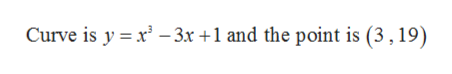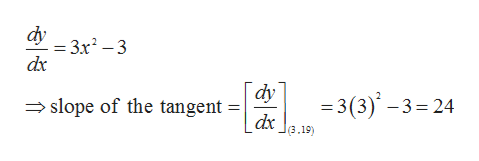Find the equation of the tangent line to the curve at a given point y=x3-3x+1 (3, 19)

Question

Find the equation of the tangent line to the curve at a given point

y=x3-3x+1 (3, 19)

Step 1

Given,help_outlineImage TranscriptioncloseCurve is y x-3x +1 and the point is (3,19) fullscreen
Step 2

Now the slope of the tangent is the derivative of the ...help_outlineImage Transcriptionclosedy = 3x2-3 dx dy =3 (3)-3 24 dx slope of the tangent (3.19) fullscreen

Want to see the full answer?

See Solution

Want to see this answer and more?

Our solutions are written by experts, many with advanced degrees, and available 24/7

See Solution
Tagged in

Derivative